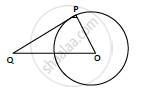Share

# Find the Length of a Tangent Drawn to a Circle with Radius 5cm, from a Point 13 Cm from the Center of the Circle. - CBSE Class 10 - Mathematics

ConceptCircles Examples and Solutions

#### Question

Find the length of a tangent drawn to a circle with radius 5cm, from a point 13 cm from the center of the circle.

#### Solution

Consider a circle with center O.

OP = radius = 5 cm.

A tangent is drawn at point P, such that line through O intersects it at Q, OB = 13cm.

Length of tangent PQ = ?A + P, we know that tangent and radius are perpendicular.

Δ𝑂𝑃𝑄 is right angled triangle, ∠OPQ = 90°

𝐵𝑦 𝑝𝑦𝑡ℎ𝑎𝑔𝑜𝑟𝑎𝑠 𝑡ℎ𝑒𝑜𝑟𝑒𝑚, 𝑂𝑄2 = 𝑂𝑃2 + 𝑃𝑄2

⇒ 132 = 52 + 𝑃𝑄2

⇒ 𝑃𝑄2 = 169 − 25 = 144

⇒ PQ = sqrt(144) = 12𝑐𝑚

Length of tangent = 12 cm

Is there an error in this question or solution?

#### Video TutorialsVIEW ALL 

Solution Find the Length of a Tangent Drawn to a Circle with Radius 5cm, from a Point 13 Cm from the Center of the Circle. Concept: Circles Examples and Solutions.
S